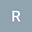The Historical and Mathematical Contributions from                                                              Fibonacci
•• Rikki
Rikki

Corresponding Author:rikki_94@yahoo.com

Author Profile## Abstract

Fibonacci is a widely known medieval Italian  mathematician who made some major contributions to mathematics. He wrote numerous books that all contributed to the extension of mathematical concepts of other well known mathematicians as well as creating new contributions towards mathematics. He is most famous for his Fibonacci sequence and numbers as well as the incredible patterns that begin to evolve. The numbers in his sequence are manifested through numerous applications towards mathematics that include number theory too. A couple of further mathematical applications seen within the Fibonacci numbers and sequence include how these numbers are represented in the Euclidean Algorithm and how we can use Fibonacci numbers to convert from miles to kilometers.